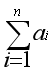Problem F: Combination plus

## Problem F: Combination plus

Time Limit: 2 Sec  Memory Limit: 512 MB
Submit: 357  Solved: 69
[Submit][Status][Web Board]

## Description

There are n sorted values a1……an, you are asked to combine them to one, you are allowed to combine at most k values to one each time, and the cost of one combination is the sum of all values that you combine, the cost are not allowed to be more than m, you are asked to find the minimum k.

## Input

The first line will be an integer T, which is the number of test cases. (1 <= T <= 10). For each test case, the first line will be two integer n(1 <= n <= 105) and m(<= m <= 1018), n is the number of values, m is the limit of cost, the second line will be n integers, a1……an(1 <= ai <= 1.2*109). We promise that ai >= ai-1 (2 <= i <= n)

## Output

For each test output the minimum k.

## Sample Input

1
2 100
1 2

## Sample Output

2

[Submit][Status]